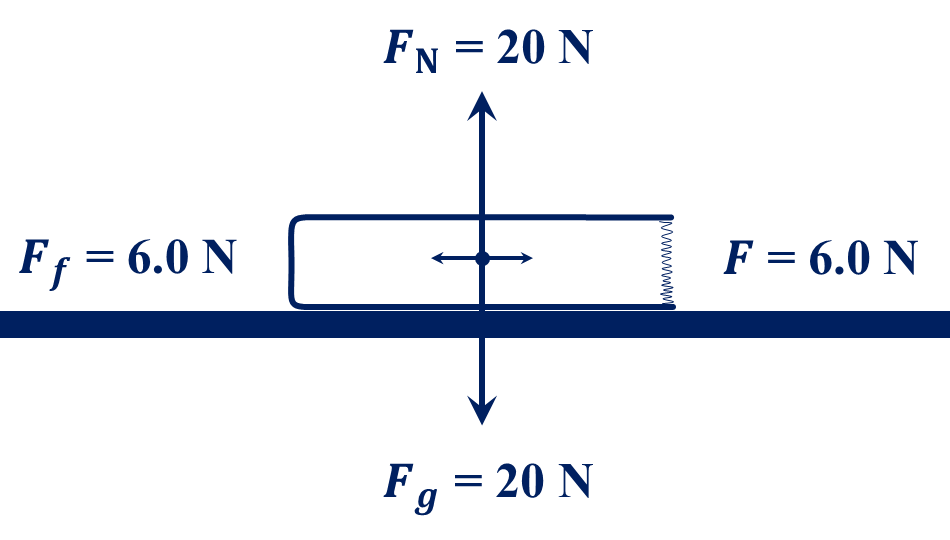﻿ Week 8 Doc O's FHS Physics Assignment Page

Week 8:  25 October to 29 October 2021

25 October 2019:In Class:  Force of Gravity, Normal Force, Weight, and Friction.

Expectations:  Students will solve simple friction problems.

Due:  Pages 1:161-163 (202 – 211).

Homework:  Pages 1:163-164 (212 – 217).

26 October 2019:

In Class:  Relationship between Weight and Friction:

• Ff  =  μ FN
• Ff  = force of friction
μ = coefficient of friction
FN = normal force
• Expectations:  Students will solve friction problems.

Due:  Pages 1:163-164 (212 – 217).

Homework:  Pages 1:164-165 (218 – 227).27 October 2019:

In Class:  Forces on a Ramp (free body diagrams).

Expectations:  Students will resolve and solve friction and free body diagram problems involving ramps.

Due:  Pages 1:164-165 (218 – 227).

Homework:  Pages 1:165-167 (228 – 236).

28 October 2019:

No Class:  Physics does not meet on Thursday.

29 October 2019:

In Class:  Momentum:

• p = m v
• p = momentum
m = mass
v = velocity or speed
• Impulse:

• J = Fnet t = Δp
• J = impulse
Fnet = net force
t = time
Δ = change
p = momentum
• Expectations:  Students will solve momentum and impulse problems.

Lab 3:
Data collection and analysis of Speeding Cars Lab.

Expectations:  Students will be able to use a spreadsheet to analyze distance and time data and to create graphs to find velocity.

Due:  Pages 1:165-167 (228 – 236).

Homework:  Pages 1:167-169 (237 – 252).

Note:  1st quarter ends 12 November 2021.